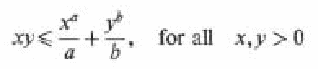Pathways To Learning... Since 2005 Hong Kong Registered School 566985

# XYZ - x-axis to Z-score - Mathematics Dictionary

A   B   C   D   E   F   G   H   I   J   K   L   M   N   O   P   Q   R   S   T   U   V   W   X   Y   Z

## X

x-axis: The line formed by the collection of points where all variables other than x is set to zero. (And different points are obtained by setting x to be different values.) It is used as (one of) the reference objects in specifying position in the rectilinear (Cartesian) coordinates system.

x-coordinate: Known as the abscissa in the old days. It measures the signed distance (i.e. displacement) of the point from the line/plane/hyper-plane y=0. It is one component in the determination of the position of a point in the rectilinear (Cartesian) coordinates system.

## Y

yard: An Imperial British unit of length defined to be exactly three feet.

y-axis: Similar to the x-axis, it is the line formed from points all of whose coordinates, other than y, is set to zero. In 2-dimensions, together with the x-axis, they form the reference from which all other positions are described.

y-coordinate: Known as the ordinate in the old days. It measures the signed distance (i.e. displacement) of the point from the line/plane/hyper-plane y=0. It is one component in the determination of the position of a point in the rectilinear (Cartesian) coordinates system.

year: A unit of time based on the period of the Earth's orbit around the Sun.

Precisely how long a year is depends on the definition and the method of measurement, giving rise to different concepts such as the many different types of astronomical years, as well as a calendar year.

For calendar years, the rule is to have an extra day is February (i.e. the 29th) every four years. So as to prevent the tiny difference between a calendar year and a sidereal year from allowing them to drift too far apart. Although since a sidereal year is not exactly 365.25 solar days, there are addtional rules that govern the placement of leap years on top of the "multiple of four" rule. For example, despite being a multiple of 4, years that are multiples of 100 would not be a leap year (this is why the year 1900 was not a leap year), and yet, if it is a multiple of 400, then it is a leap year again (and this is why the year 2000 was still a leap year.).

Young's inequality: An inequality class="d-title" named for English mathematician William Henry Young. It states that for a and b positive such that 1/a + 1/b = 1, thenNote that given the equality that a and b have to satify, 0 < a < 1 would mean that 1/a > 1, would in turn dictates that b be negative. Since neither is allowed to be negative, by the contrapositive of the above statement, a and b cannot be less than or equal 1. Hence, given the other conditions, stating that a and b must be positive amounts to the same effect as stating that a and b have to be larger than 1.

## Z

Z: The set of integers, Often appear bold in printed matter and written with Blackboard-Bold font. The letter Z is used because that is the first letter of the German word Zahlen (i.e. numbers).

Z+: The set of all positive integers. This is also known as the natural number, although some authors prefer this due to the ambiguity of the natural numbers. (i.e. the two definitions of the natural numbers.)

z-axis: The axis representing values of the variable z, usually the "third" dimension in rectilinear (i.e. Cartesian ) coordinates systems, as well as Cylindrical Polar Coordinates.

z-coordinate: The ordinate representing values of the variable z, usually the "third" dimension in rectilinear (i.e. Cartesian) coordinates systems, as well as Cylindrical Polar coordinates.

zero: 1. The numeral 0, intuitively thought of as representing nothing. It is importnt to point out that it is still a number. So that, it represents nothing rather than it "IS" nothing.

2. (of a function): A zero of a function is also known as the root of a function, that is, an argument that produces a value of zero for the function.

zero angle: An "angle" of zero degrees, in the definition of angles extended to cover what is usually not thoguht of as angles at all. The straight angle (180 degrees) is another such definition. It is mostly used for uniformity in the theorems and definitions that also involves "traditional" angles. Also known as the null angle.

zero matrix: The identity element of matrix addition (i.e. a matrix which, when added, does not change the other matrix addend.) and incidentally, the annihilator of matrix multiplication. (i.e. a matrix which, when multiplied, both on the left and on the right, does not change the other matrix multiplier/multiplicand.) All elements of this matrix are zero and it is also known as the null matrix.

zero vector: The vector with zero magnitude and "no directions". It is the identity element in the operation of vector addition.

Z-score: The argument for the probability density function of the normal distribution. Since the standard normal distribution (Z) has mean zero and standard deviation one, it can also be thought of as the number of standard deviations away from the mean.

A   B   C   D   E   F   G   H   I   J   K   L   M   N   O   P   Q   R   S   T   U   V   W   X   Y   Z

Genius is one percent inspiration and ninety-nine percent perspiration.